Next: Monte Carlo Integration Up: A Review of Monte Previous: Derivation of the Rendering

# Quasi-Random Walk Methods

In equation 15 the unknown function I appears on both sides. Substituting the right side's I by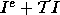, which is obviously I according to the equation, we get: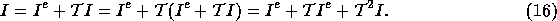Repeating this step n times, the original equation can be expanded into a Neumann series: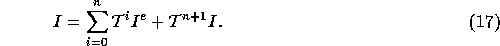If integral operator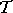is contractive, that is if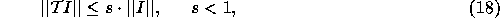then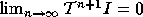, thus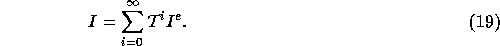The contractive property ofcomes from the fact that a reflection or refraction always decreases the energy.

The terms of this infinite Neumann series have intuitive meaning as well: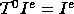comes from the emission,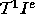comes from a single reflection,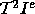from two reflections, etc.

In order to study the structure of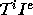, let us consider the case of i=2: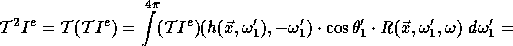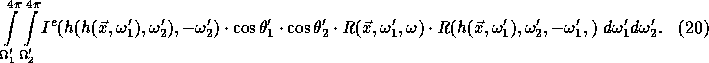To evaluate the integrand at point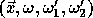, the following algorithm must be executed:

1. Surface point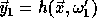-- that is the point which is visible from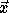at direction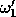-- must be determined. This can be done by sending a ray frominto directionand indentifying the surface that is first intersected.
2. Surface point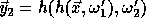-- that is the point visible from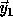at direction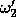is identified. This means the continuation of the ray tracing at direction.
3. The emission intensity of the surface at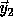in the direction of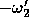is acquired and is multiplied with the cosine terms and the BRDFs of the two reflections.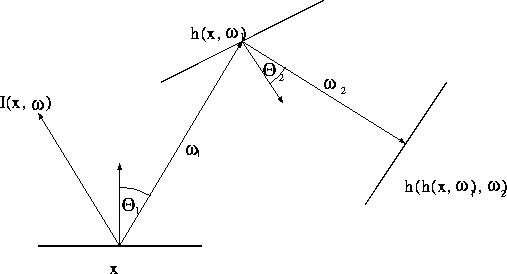Figure: The integrand of the second bound is a two-step walk

This algorithm can easily be generalised for arbitrary number of reflections. In order to compute the contribution of the nth reflection of the light to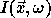, the following function should be integrated: A ray is emanated recursively fromat directionthen from the found surface at, etc. until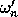. The emission intensity at the end of the walk is read and multiplied by the BRDFs and the cosine terms of the stages of the walk.

These walks provide the value of the integrand at ``point''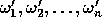. The integral is estimated as the average of these values. Directionsshould be selected to minimise the error of the approximation.

Thus we can conclude that the solution of the rendering equation requires the evaluation of high-dimensional ingegrals.Next: Monte Carlo Integration Up: A Review of Monte Previous: Derivation of the Rendering

Csébfalvi Balázs
Tue Apr 15 18:39:13 METDST 1997